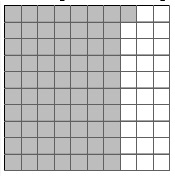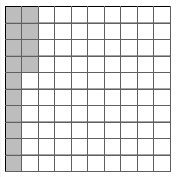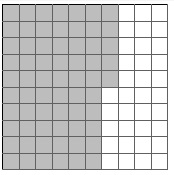# Finding the Percentage of a Grid that is Shaded

In this lesson, we usually are given 10 × 10 grids having 100 squares. Some of these squares are shaded. We are asked to find the percentage of squares that are shaded.

Rule for finding the percentage of shaded squares

• First the shaded squares are counted and the number of such squares is found.

• This number of shaded squares is out 100 squares in the 10 × 10 grid.

• Therefore, by definition of a percentage, this number itself is the percentage of shaded squares in the grid.

For example, if the number of shaded squares in a 10 × 10 grid is say 18, then the percentage of shaded squares in the grid is 18%.

Write the percentage of the shaded squares for given grid### Solution

Step 1:

Given is a 10 × 10 grid having 100 squares.

The number of shaded squares gives the percentage of the grid as that is out of a total of 100 squares.

Step 2:

The number of shaded squares is 71. Therefore, the percentage of the shaded squares is 71%

Write the percentage of the shaded squares for given grid### Solution

Step 1:

Given is a 10 × 10 grid having 100 squares.

The number of shaded squares gives the percentage of the grid as that is out of a total of 100 squares.

Step 2:

The number of shaded squares is 14. Therefore, the percentage of the shaded squares is 14%

Write the percentage of the shaded squares for given grid### Solution

Step 1:

Given is a 10 × 10 grid having 100 squares.

The number of shaded squares gives the percentage of the grid as that is out of a total of 100 squares.

Step 2:

The number of shaded squares is 65. Therefore, the percentage of the shaded squares is 65%